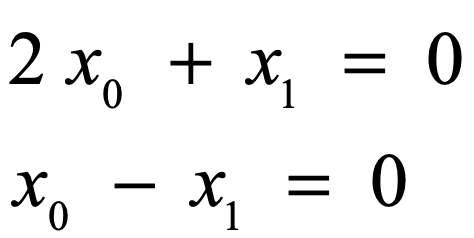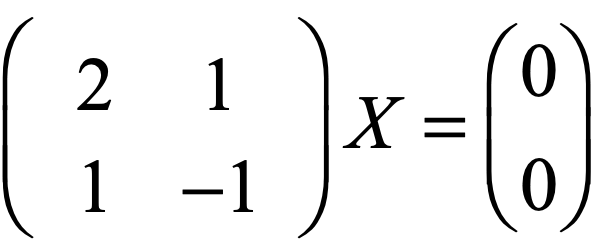# Write a solver for systems of equations by using Lapack on Mac OS

Apple provides the Accelerate library which includes the linear algebra library (Lapack). In this post, we will go through writing a simple C++ program to use this library on Mac OS.

Let’s solve the following equations:We can rewrite the equations as:Now, let’s write our code:

Now, run the code by using the following commands in a terminal:

Tags:

Categories:

Updated: